http www comp nus edu sgcs 1010 UNIT

• Slides: 38http: //www. comp. nus. edu. sg/~cs 1010/ UNIT 9 Multidimensional ArraysAaron Tan, NUS Multidimensional Arrays Unit 9 - 2 Unit 9: Multidimensional Arrays Objective: § Understand the concept of multi-dimensional § arrays Problem solving using multidimensional arrays Reference: § Chapter 7: Array Pointers § Section 7. 8 Multidimensional Arrays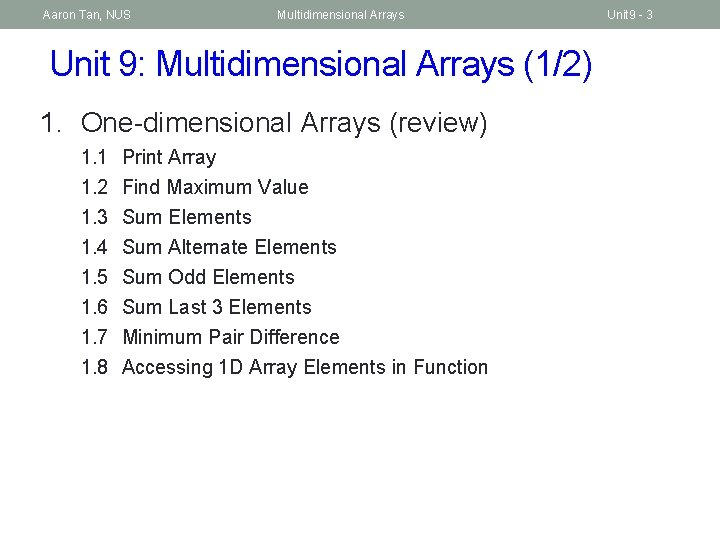Aaron Tan, NUS Multidimensional Arrays Unit 9: Multidimensional Arrays (1/2) 1. One-dimensional Arrays (review) 1. 1 Print Array 1. 2 1. 3 1. 4 1. 5 1. 6 1. 7 1. 8 Find Maximum Value Sum Elements Sum Alternate Elements Sum Odd Elements Sum Last 3 Elements Minimum Pair Difference Accessing 1 D Array Elements in Function Unit 9 - 3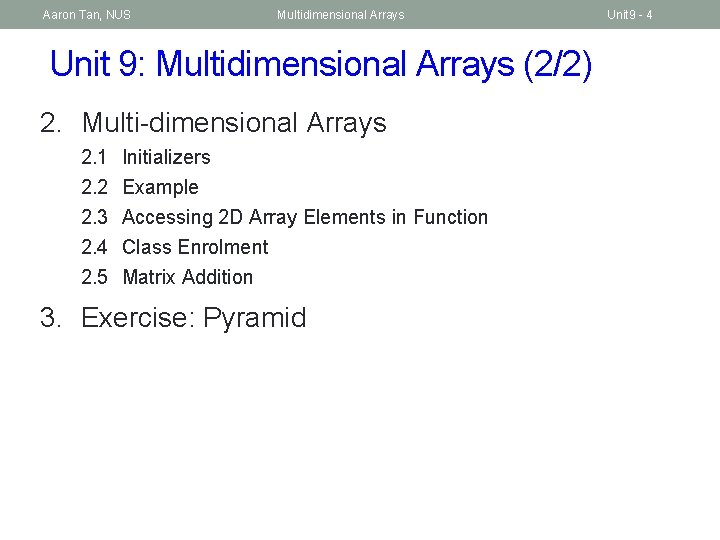Aaron Tan, NUS Multidimensional Arrays Unit 9: Multidimensional Arrays (2/2) 2. Multi-dimensional Arrays 2. 1 Initializers 2. 2 2. 3 2. 4 2. 5 Example Accessing 2 D Array Elements in Function Class Enrolment Matrix Addition 3. Exercise: Pyramid Unit 9 - 4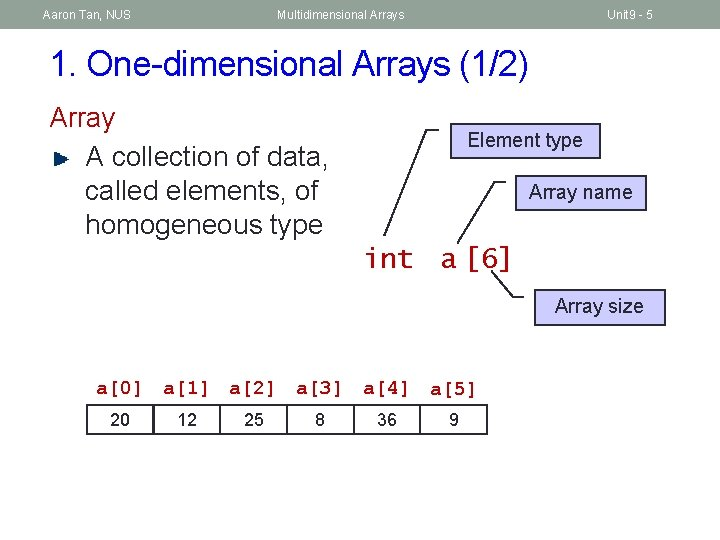Aaron Tan, NUS Multidimensional Arrays Unit 9 - 5 1. One-dimensional Arrays (1/2) Array A collection of data, called elements, of homogeneous type Element type Array name int a  Array size a a a a a a 20 12 25 8 36 9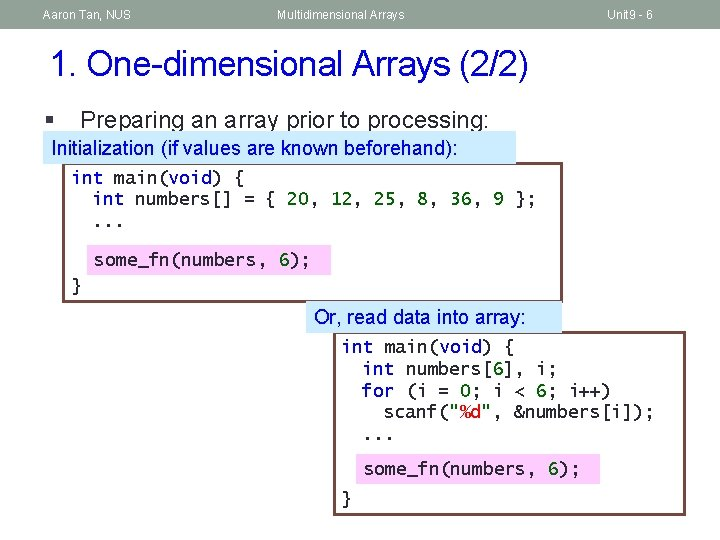Aaron Tan, NUS Multidimensional Arrays Unit 9 - 6 1. One-dimensional Arrays (2/2) § Preparing an array prior to processing: Initialization (if values are known beforehand): int main(void) { int numbers[] = { 20, 12, 25, 8, 36, 9 }; . . . some_fn(numbers, 6); } Or, read data into array: int main(void) { int numbers, i; for (i = 0; i < 6; i++) scanf("%d", &numbers[i]); . . . some_fn(numbers, 6); }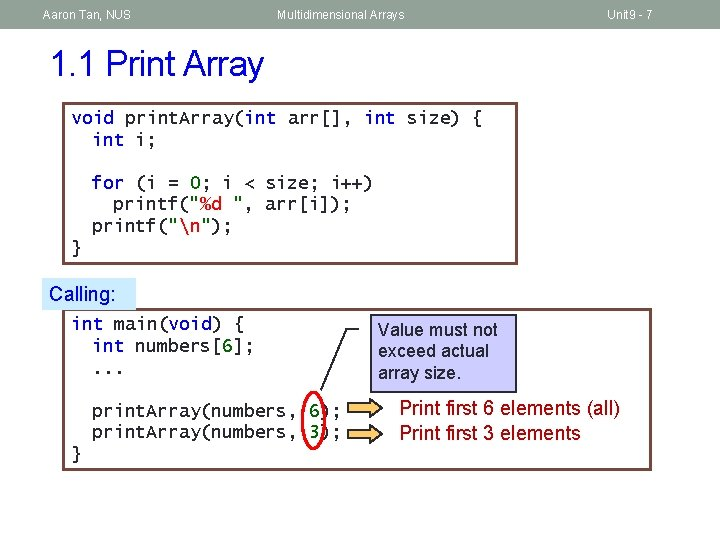Aaron Tan, NUS Multidimensional Arrays Unit 9 - 7 1. 1 Print Array void print. Array(int arr[], int size) { int i; for (i = 0; i < size; i++) printf("%d ", arr[i]); printf("n"); } Calling: int main(void) { int numbers; . . . print. Array(numbers, 6); print. Array(numbers, 3); } Value must not exceed actual array size. Print first 6 elements (all) Print first 3 elements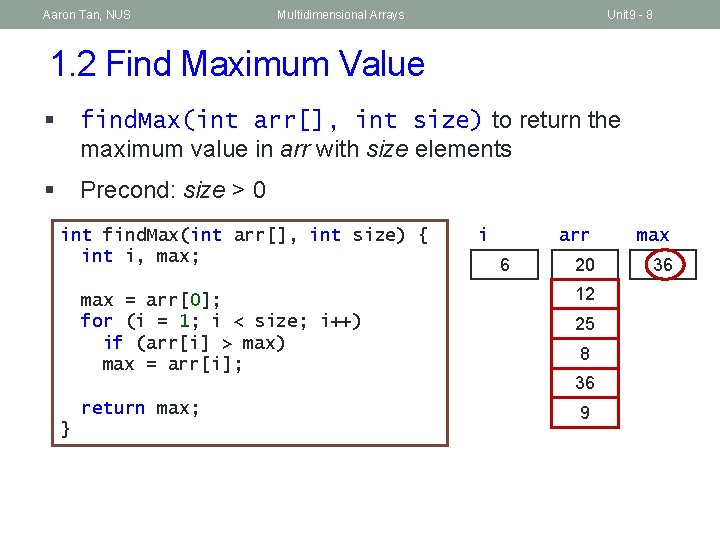Aaron Tan, NUS Multidimensional Arrays Unit 9 - 8 1. 2 Find Maximum Value § find. Max(int arr[], int size) to return the maximum value in arr with size elements § Precond: size > 0 int find. Max(int arr[], int size) { int i, max; } i 1 5 4 3 2 6 arr max 20 36 25 20 max = arr; for (i = 1; i < size; i++) if (arr[i] > max) max = arr[i]; 12 return max; 9 25 8 36Aaron Tan, NUS Multidimensional Arrays Unit 9 - 9 Computational Thinking time again! 1. 3 Sum Elements § sum(int arr[], int size) to return the sum of elements in arr with size elements § Precond: size > 0 int sum(int arr[], int size) { int i, sum = 0; for (i = 0; i < size; i++) sum += arr[i]; return sum; } i arr 3 2 1 0 4 5 6 20 12 25 8 36 9 sum 101 110 57 32 20 65 0Aaron Tan, NUS Multidimensional Arrays Unit 9 - 10 Computational Thinking time again! 1. 4 Sum Alternate Elements § sum. Alt(int arr[], int size) to return the sum of alternate elements (1 st, 3 rd, 5 th, etc. ) § Precond: size > 0 int sum. Alt(int sum(int arr[], intint size) { { int i, sum = 0; for (i = 0; i < size; i+=2) i++) sum += arr[i]; return sum; } i 6 4 2 0 arr sum 20 81 45 20 0 12 25 8 36 9Aaron Tan, NUS Multidimensional Arrays Unit 9 - 11 Computational Thinking time again! 1. 5 Sum Odd Elements § sum. Odd(int arr[], int size) to return the sum of elements that are odd numbers § Precond: size > 0 int sum. Odd(int arr[], size) sum(int arr[], intint size) { { int i, sum = 0; for (i = 0; i < size; i++) if == 1) sum(arr[i]%2 += arr[i]; sum += arr[i]; return sum; } i 5 4 3 2 1 0 6 arr sum 20 25 34 0 12 25 8 36 9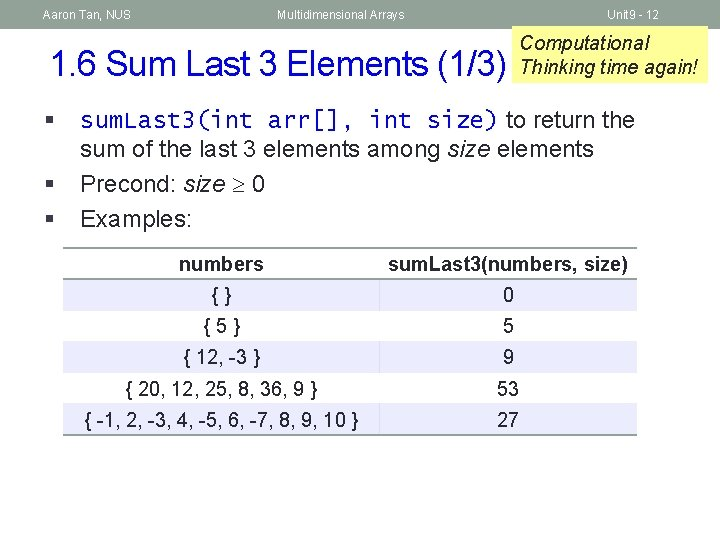Aaron Tan, NUS Multidimensional Arrays Unit 9 - 12 1. 6 Sum Last 3 Elements (1/3) § § § Computational Thinking time again! sum. Last 3(int arr[], int size) to return the sum of the last 3 elements among size elements Precond: size 0 Examples: numbers sum. Last 3(numbers, size) {} 0 {5} 5 { 12, -3 } 9 { 20, 12, 25, 8, 36, 9 } 53 { -1, 2, -3, 4, -5, 6, -7, 8, 9, 10 } 27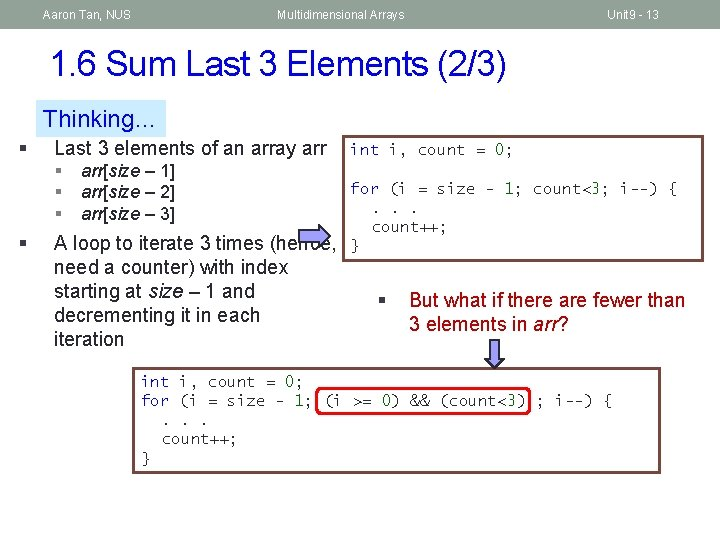Aaron Tan, NUS Multidimensional Arrays Unit 9 - 13 1. 6 Sum Last 3 Elements (2/3) Thinking… § Last 3 elements of an array arr § arr[size – 1] § arr[size – 2] § arr[size – 3] § A loop to iterate 3 times (hence, need a counter) with index starting at size – 1 and decrementing it in each iteration int i, count = 0; for (i = size - 1; count<3; i--) {. . . count++; } § But what if there are fewer than 3 elements in arr? int i, count = 0; for (i = size - 1; (i >= 0) && (count<3) ; i--) {. . . count++; }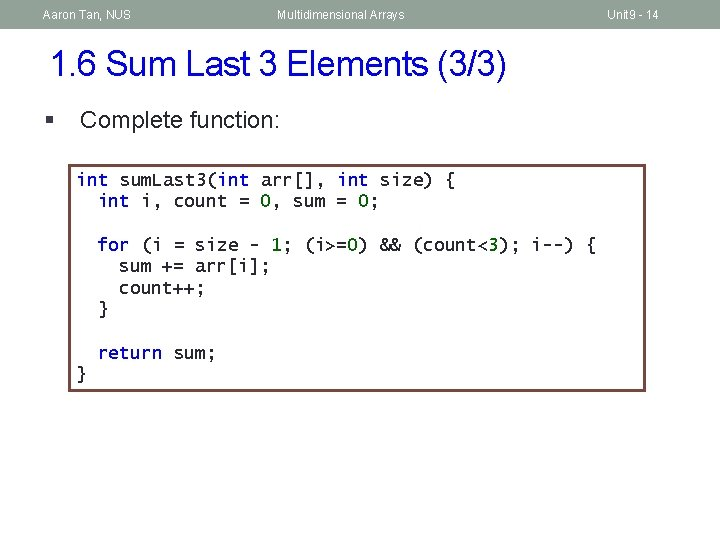Aaron Tan, NUS Multidimensional Arrays 1. 6 Sum Last 3 Elements (3/3) § Complete function: int sum. Last 3(int arr[], int size) { int i, count = 0, sum = 0; for (i = size - 1; (i>=0) && (count<3); i--) { sum += arr[i]; count++; } return sum; } Unit 9 - 14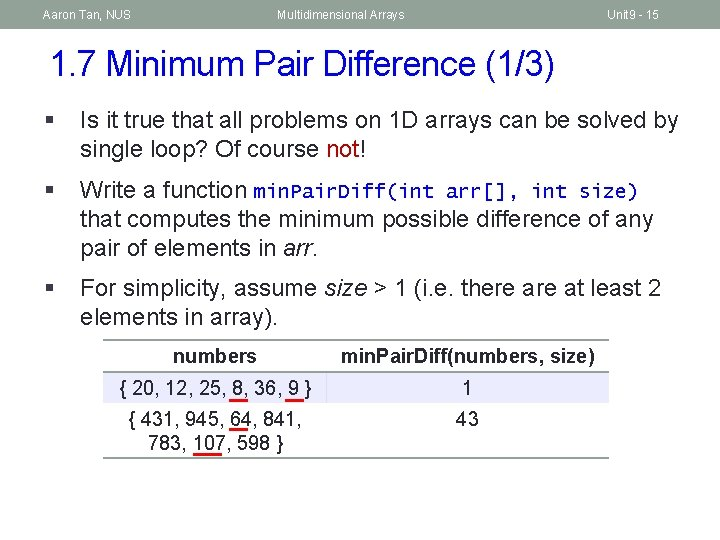Aaron Tan, NUS Multidimensional Arrays Unit 9 - 15 1. 7 Minimum Pair Difference (1/3) § Is it true that all problems on 1 D arrays can be solved by single loop? Of course not! § Write a function min. Pair. Diff(int arr[], int size) that computes the minimum possible difference of any pair of elements in arr. § For simplicity, assume size > 1 (i. e. there at least 2 elements in array). numbers min. Pair. Diff(numbers, size) { 20, 12, 25, 8, 36, 9 } 1 { 431, 945, 64, 841, 783, 107, 598 } 43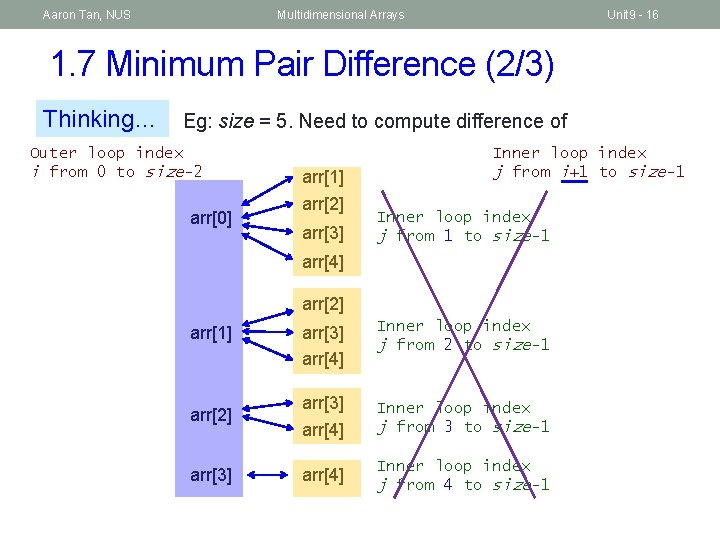Aaron Tan, NUS Multidimensional Arrays Unit 9 - 16 1. 7 Minimum Pair Difference (2/3) Thinking… Eg: size = 5. Need to compute difference of Outer loop index i from 0 to size-2 arr arr arr arr Inner loop index j from i+1 to size-1 Inner loop index j from 1 to size-1 arr arr arr arr Inner loop index j from 2 to size-1 arr arr arr Inner loop index j from 3 to size-1 arr arr Inner loop index j from 4 to size-1 arrAaron Tan, NUS Multidimensional Arrays Unit 9 - 17 1. 7 Minimum Pair Difference (3/3) The code… Outer loop index i from 0 to size-2 Inner loop index j from i+1 to size-1 int min. Pair. Diff(int arr[], int size) { int i, j, diff, min. Diff; min. Diff = abs(arr – arr); // init min diff. for (i = 0; i < size-1; i++) for (j = i+1; j < size; j++) { diff = abs(arr[i] – arr[j]); if (diff < min. Diff) § This kind of nested loop is found in many min. Diff = diff; applications involving 1 D array, for } example, sorting (to be covered later). return min. Diff; } § In fact, this problem can be solved by first sorting the array, then scan through the array once more to pick the pair of neighbours with the smallest difference.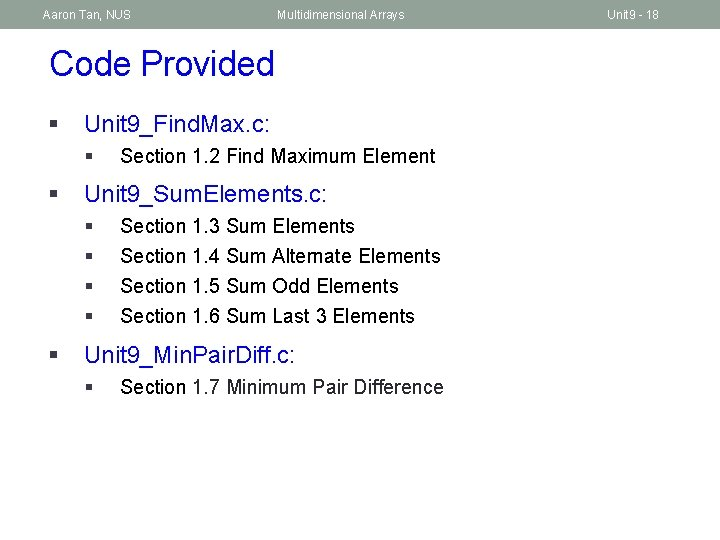Aaron Tan, NUS Multidimensional Arrays Code Provided § Unit 9_Find. Max. c: § § Unit 9_Sum. Elements. c: § § § Section 1. 2 Find Maximum Element Section 1. 3 Sum Elements Section 1. 4 Sum Alternate Elements Section 1. 5 Sum Odd Elements Section 1. 6 Sum Last 3 Elements Unit 9_Min. Pair. Diff. c: § Section 1. 7 Minimum Pair Difference Unit 9 - 18Aaron Tan, NUS Multidimensional Arrays Unit 9 - 19 1. 8 Accessing 1 D Array Elements in Function (1/2) A function header with array parameter, int sum(int a[ ], int size) § A value is not necessary (and is ignored by compiler if provided) as accessing a particular array element requires only the following information § § § Why is it not necessary to have a value in here to indicate the “real” size? The address of the first element of the array The size of each element Both information are known § For example, when the above function is called with ans = sum(numbers, 6); in the main(), the address of the first element, &numbers, is copied into the parameter a § The size of each element is determined since the element type (int) is given (in sunfire, an integer takes up 4 bytes)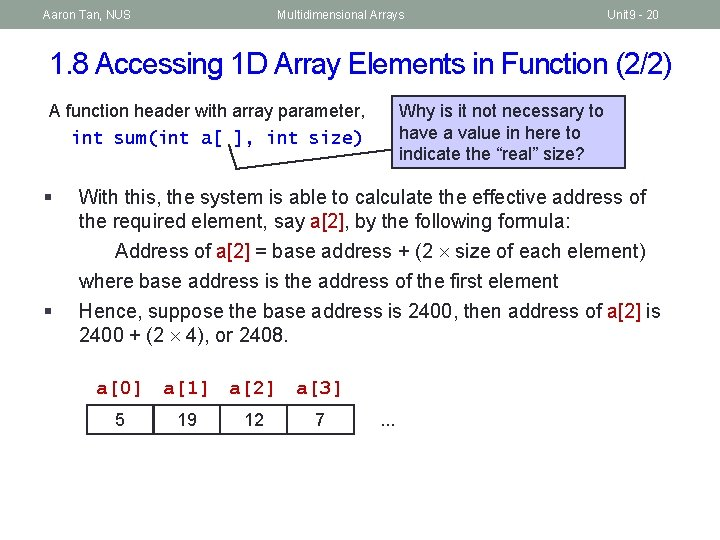Aaron Tan, NUS Multidimensional Arrays Unit 9 - 20 1. 8 Accessing 1 D Array Elements in Function (2/2) Why is it not necessary to have a value in here to indicate the “real” size? A function header with array parameter, int sum(int a[ ], int size) § § With this, the system is able to calculate the effective address of the required element, say a, by the following formula: Address of a = base address + (2 size of each element) where base address is the address of the first element Hence, suppose the base address is 2400, then address of a is 2400 + (2 4), or 2408. a a a a 5 19 12 7 . . .Aaron Tan, NUS Multidimensional Arrays Unit 9 - 21 2. Multi-dimensional Arrays (1/2) § In general, an array can have any number of dimensions § Example of a 2 -dimensional (2 D) array: // array with 3 rows, 5 columns int a; a = 2; a = 9; a = a + 7; § 0 1 2 3 4 0 2 1 16 2 9 Arrays are stored in row-major order § That is, elements in row 0 comes before row 1, etc. a … row 0 a a … row 1 a a … row 2 aAaron Tan, NUS Multidimensional Arrays Unit 9 - 22 2. Multi-dimensional Arrays (2/2) § Examples of applications: 1 2 3 Jan 32. 1 31. 8 31. 9 Feb 32. 6 33. 0 31. 8 32. 3 30. 9 : matrix Dec 31 32. 3 32. 4 0 0 31. 6 32. 2 Daily temperatures: temperatures Suise Jerna Zass Emily 30 Ex 1 Ex 2 Ex 3 59 68 60 0 Ex 1 Ex 2 Lab 1 76 80 Ex 3 Lab 1 62 Lab 2 60 72 48 Lab 3 67 71 75 Lab 3 76 80 38 52 35 Lab 4 60 72 62 Lab 4 48 Lab 5 78 86 82 Lab 5 58 79 73 Ex 1 Ex 2 Ex 3 Lab 1 79 75 66 Lab 2 90 83 77 Lab 3 81 73 79 Lab 4 58 64 52 Lab 5 93 80 85 Ex 1 Ex 2 Ex 3 Lab 1 52 50 45 Lab 2 57 60 63 Lab 3 52 59 66 Lab 4 33 42 37 Lab 5 68 68 72 Students’ lab marks: marks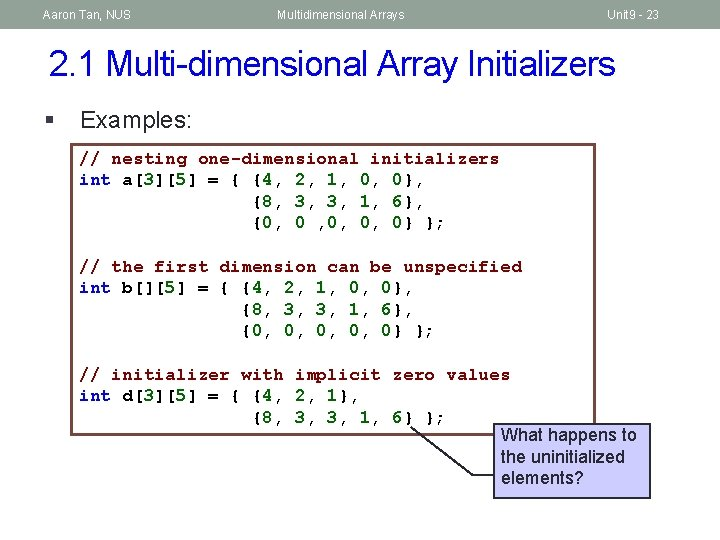Aaron Tan, NUS Multidimensional Arrays Unit 9 - 23 2. 1 Multi-dimensional Array Initializers § Examples: // nesting one-dimensional initializers int a = { {4, 2, 1, 0, 0}, {8, 3, 3, 1, 6}, {0, 0 , 0, 0, 0} }; // the first dimension can be unspecified int b[] = { {4, 2, 1, 0, 0}, {8, 3, 3, 1, 6}, {0, 0, 0} }; // initializer with implicit zero values int d = { {4, 2, 1}, {8, 3, 3, 1, 6} }; What happens to the uninitialized elements?Aaron Tan, NUS Multidimensional Arrays Unit 9 - 24 2. 2 Multi-dimensional Array: Example Unit 9_2 DArray. c #include <stdio. h> #define N 5 // number of columns in array int sum. Array(int [][N], int); // function prototype int main(void) { int foo[][N] = { {3, 7, 1}, {2, 1}, {4, 6, 2} }; printf("Sum is %dn", sum. Array(foo, 3)); printf("Sum is %dn", sum. Array(foo, 2)); return 0; } Second dimension must be specified; first dimension is // To sum all elements in arr not required. int sum. Array(int arr[][N], int rows) { int i, j, total = 0; for (i = 0; i < rows; i++) { Sum is 26 for (j = 0; j < N; j++) { total += arr[i][j]; Sum is 14 } } return total; }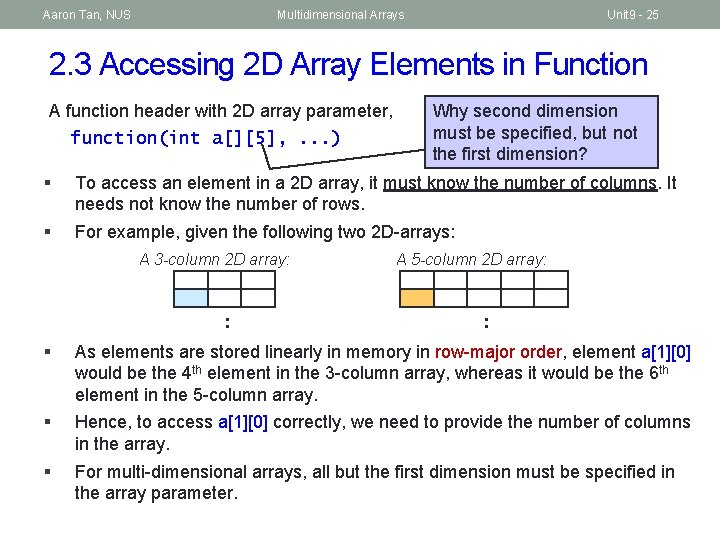Aaron Tan, NUS Multidimensional Arrays Unit 9 - 25 2. 3 Accessing 2 D Array Elements in Function A function header with 2 D array parameter, function(int a[], . . . ) Why second dimension must be specified, but not the first dimension? § To access an element in a 2 D array, it must know the number of columns. It needs not know the number of rows. § For example, given the following two 2 D-arrays: A 3 -column 2 D array: : A 5 -column 2 D array: : § As elements are stored linearly in memory in row-major order, element a would be the 4 th element in the 3 -column array, whereas it would be the 6 th element in the 5 -column array. § Hence, to access a correctly, we need to provide the number of columns in the array. § For multi-dimensional arrays, all but the first dimension must be specified in the array parameter.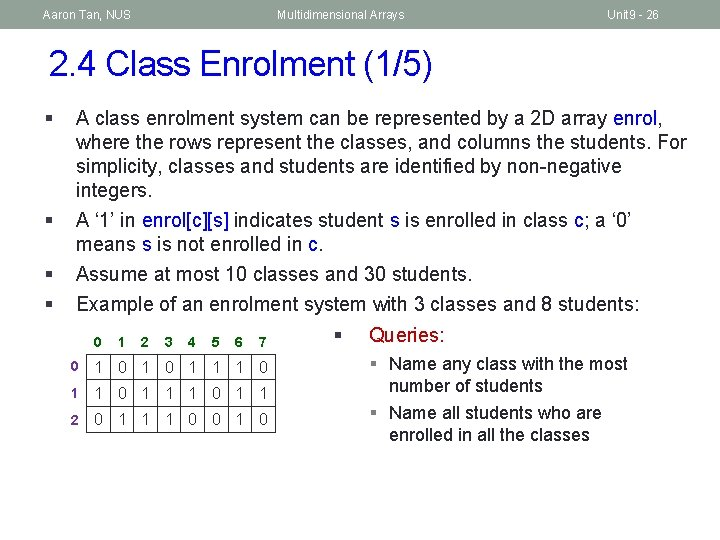Aaron Tan, NUS Multidimensional Arrays Unit 9 - 26 2. 4 Class Enrolment (1/5) § § A class enrolment system can be represented by a 2 D array enrol, where the rows represent the classes, and columns the students. For simplicity, classes and students are identified by non-negative integers. A ‘ 1’ in enrol[c][s] indicates student s is enrolled in class c; a ‘ 0’ means s is not enrolled in c. Assume at most 10 classes and 30 students. Example of an enrolment system with 3 classes and 8 students: 0 1 2 3 4 5 6 7 0 1 0 1 1 2 0 1 1 1 0 0 1 0 § Queries: § Name any class with the most number of students § Name all students who are enrolled in all the classes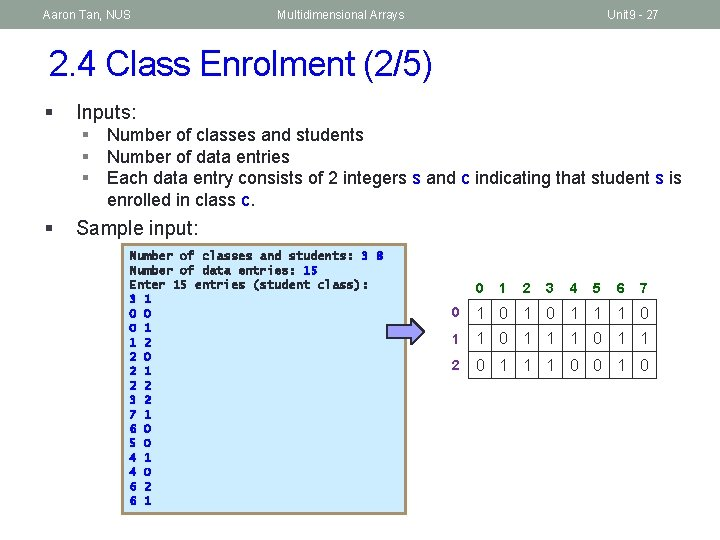Aaron Tan, NUS Multidimensional Arrays Unit 9 - 27 2. 4 Class Enrolment (2/5) § Inputs: § Number of classes and students § Number of data entries § Each data entry consists of 2 integers s and c indicating that student s is enrolled in class c. § Sample input: Number of classes and students: 3 8 Number of data entries: 15 Enter 15 entries (student class): 3 1 0 0 0 1 1 2 2 0 2 1 2 2 3 2 7 1 6 0 5 0 4 1 4 0 6 2 6 1 0 1 2 3 4 5 6 7 0 1 0 1 1 2 0 1 1 1 0 0 1 0Aaron Tan, NUS Multidimensional Arrays Unit 9 - 28 2. 4 Class Enrolment (3/5) #define MAX_CLASSES 10 #define MAX_STUDENTS 30 int main(void) { int enrol[MAX_CLASSES][MAX_STUDENTS] = { {0} }, num. Classes, num. Students; printf("Number of classes and students: "); scanf("%d %d", &num. Classes, &num. Students); read. Inputs(enrol, num. Classes, num. Students); return 0; } 3 8 15 3 1 0 0 0 1 1 2 2 0 2 1 2 2 3 2 7 1 6 0 5 0 4 1 4 0 6 2 6 1 // Read data into array enrol void read. Inputs(int enrol[][MAX_STUDENTS], int num. Classes, int num. Students) { int entries; // number of data entries int i, class, student; printf("Number of data entries: "); scanf("%d", &entries); } printf("Enter %d data entries (student class): n", entries); // Read data into array enrol for (i = 0; i < entries; i++) { 0 1 2 3 4 5 6 7 scanf("%d %d", &student, &class); enrol[class][student] = 1; 0 1 0 1 1 1 0 } 1 1 0 1 1 2 0 1 1 1 0 0 1 0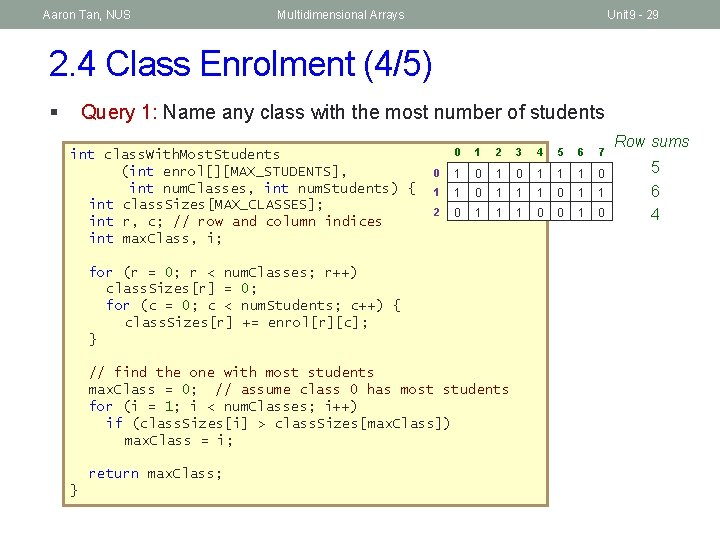Aaron Tan, NUS Multidimensional Arrays Unit 9 - 29 2. 4 Class Enrolment (4/5) § Query 1: Name any class with the most number of students int class. With. Most. Students (int enrol[][MAX_STUDENTS], int num. Classes, int num. Students) { int class. Sizes[MAX_CLASSES]; int r, c; // row and column indices int max. Class, i; 0 1 2 3 4 5 6 7 0 1 0 1 1 2 0 1 1 1 0 0 1 0 for (r = 0; r < num. Classes; r++) class. Sizes[r] = 0; for (c = 0; c < num. Students; c++) { class. Sizes[r] += enrol[r][c]; } // find the one with most students max. Class = 0; // assume class 0 has most students for (i = 1; i < num. Classes; i++) if (class. Sizes[i] > class. Sizes[max. Class]) max. Class = i; return max. Class; } Row sums 5 6 4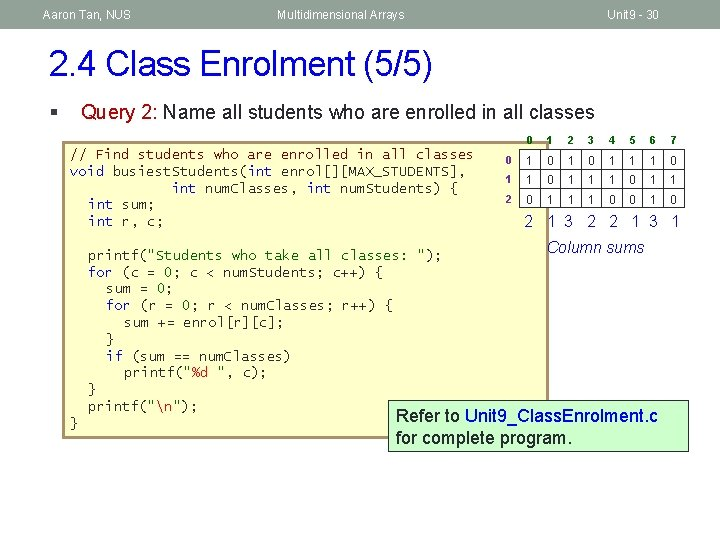Aaron Tan, NUS Multidimensional Arrays Unit 9 - 30 2. 4 Class Enrolment (5/5) § Query 2: Name all students who are enrolled in all classes // Find students who are enrolled in all classes void busiest. Students(int enrol[][MAX_STUDENTS], int num. Classes, int num. Students) { int sum; int r, c; printf("Students who take all classes: "); for (c = 0; c < num. Students; c++) { sum = 0; for (r = 0; r < num. Classes; r++) { sum += enrol[r][c]; } if (sum == num. Classes) printf("%d ", c); } printf("n"); } 0 1 2 3 4 5 6 7 0 1 0 1 1 2 0 1 1 1 0 0 1 0 2 1 3 2 2 1 3 1 Column sums Refer to Unit 9_Class. Enrolment. c for complete program.Aaron Tan, NUS Multidimensional Arrays Unit 9 - 31 2. 5 Matrix Addition (1/2) § To add two matrices, both must have the same size (same number of rows and columns). § To compute C = A + B, where A, B, C are matrices ci, j = ai, j + bi, j § Examples:Aaron Tan, NUS Multidimensional Arrays Unit 9 - 32 2. 5 Matrix Addition (2/2) Unit 9_Matrix. Ops. c // To sum mtx. A and mtx. B to obtain mtx. C void sum. Matrix(float mtx. A[][MAX_COL], float mtx. B[][MAX_COL], float mtx. C[][MAX_COL], int row_size, int col_size) { int row, col; for (row=0; row<row_size; row++) for (col=0; col<col_size; col++) mtx. C[row][col] = mtx. A[row][col] + mtx. B[row][col]; } 10 21 7 9 4 14 5 6 + 3 7 18 20 6 5 8 15 = 13 ? 28 ? 25 ? 29 ? 10 ? 11 ? 22 ? 20 ?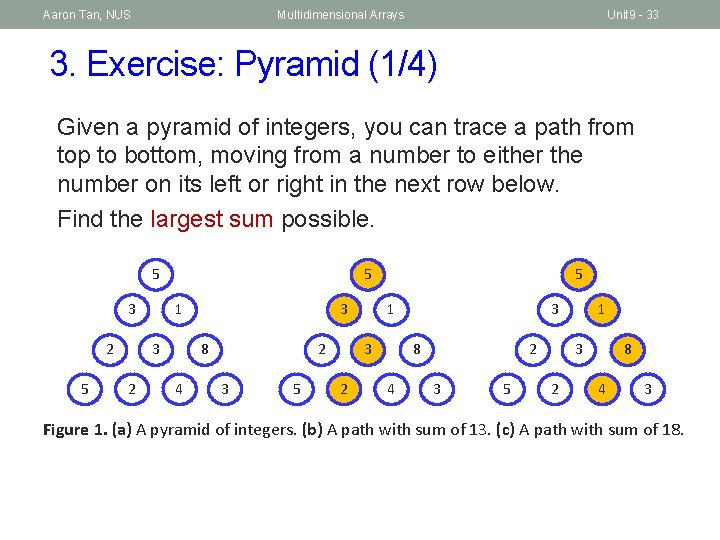Aaron Tan, NUS Multidimensional Arrays Unit 9 - 33 3. Exercise: Pyramid (1/4) Given a pyramid of integers, you can trace a path from top to bottom, moving from a number to either the number on its left or right in the next row below. Find the largest sum possible. 5 3 2 5 5 1 3 2 3 8 4 2 3 5 5 3 2 3 1 8 4 2 3 5 1 3 2 8 4 3 Figure 1. (a) A pyramid of integers. (b) A path with sum of 13. (c) A path with sum of 18.Aaron Tan, NUS Multidimensional Arrays Unit 9 - 34 3. Exercise: Pyramid (2/4) Unit 9_Pyramid. c #include <stdio. h> #define MAX_ROWS 10 int max. Path. Value(int [][MAX_ROWS], int); int scan. Triangular. Array(int [][MAX_ROWS]); void print. Triangular. Array(int [][MAX_ROWS], int); int main(void) { int size; // number of rows in the pyramid int table[MAX_ROWS]; size = scan. Triangular. Array(table); // print. Triangular. Array(table, size); // for checking printf("Maximum path value = %dn", max. Path. Value(table, size)); return 0; }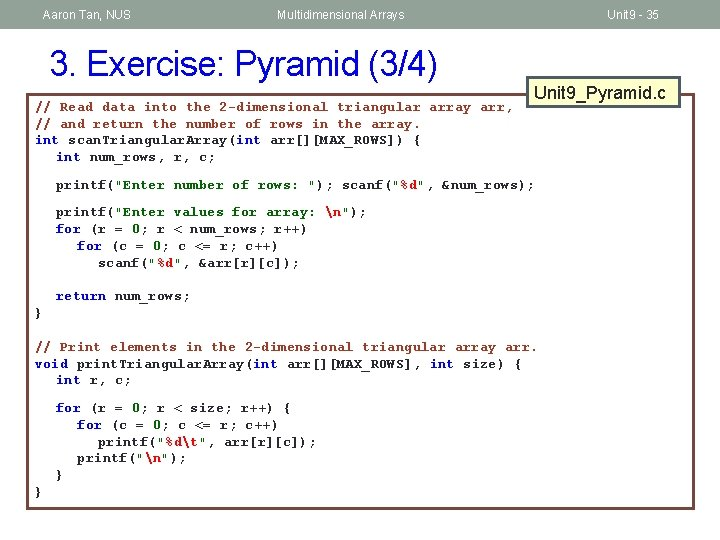Aaron Tan, NUS Multidimensional Arrays 3. Exercise: Pyramid (3/4) // Read data into the 2 -dimensional triangular array arr, // and return the number of rows in the array. int scan. Triangular. Array(int arr[][MAX_ROWS]) { int num_rows, r, c; Unit 9 - 35 Unit 9_Pyramid. c printf("Enter number of rows: "); scanf("%d", &num_rows); printf("Enter values for array: n"); for (r = 0; r < num_rows; r++) for (c = 0; c <= r; c++) scanf("%d", &arr[r][c]); return num_rows; } // Print elements in the 2 -dimensional triangular array arr. void print. Triangular. Array(int arr[][MAX_ROWS], int size) { int r, c; for (r = 0; r < size; r++) { for (c = 0; c <= r; c++) printf("%dt", arr[r][c]); printf("n"); } }Aaron Tan, NUS Multidimensional Arrays 3. Exercise: Pyramid (4/4) Unit 9 - 36 Unit 9_Pyramid. c // Compute the maximum path sum. int max. Path. Value(int arr[][MAX_ROWS], int size) { return 123; }Aaron Tan, NUS Multidimensional Arrays Summary n In this unit, you have learned about n Declaring 2 D arrays n Using 2 D arrays in problem solving Unit 9 - 37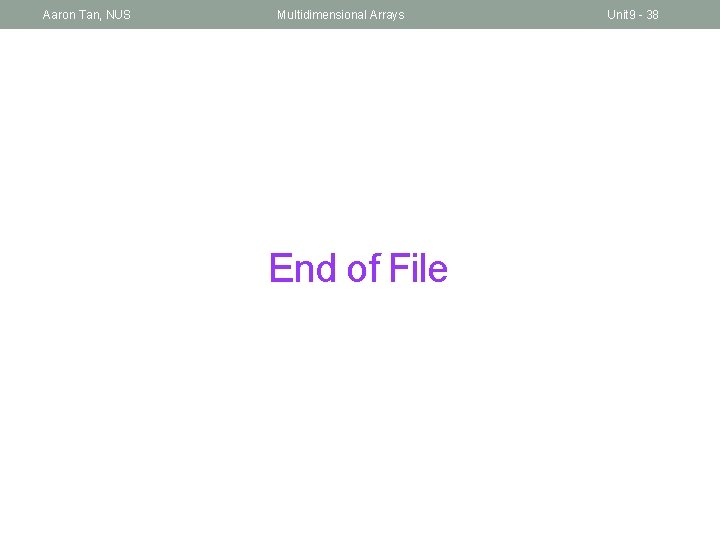Aaron Tan, NUS Multidimensional Arrays End of File Unit 9 - 38